### Project Notes

Exploring the tips and traps involved in getting accurate readings from the LTC2400 24-bit ADC.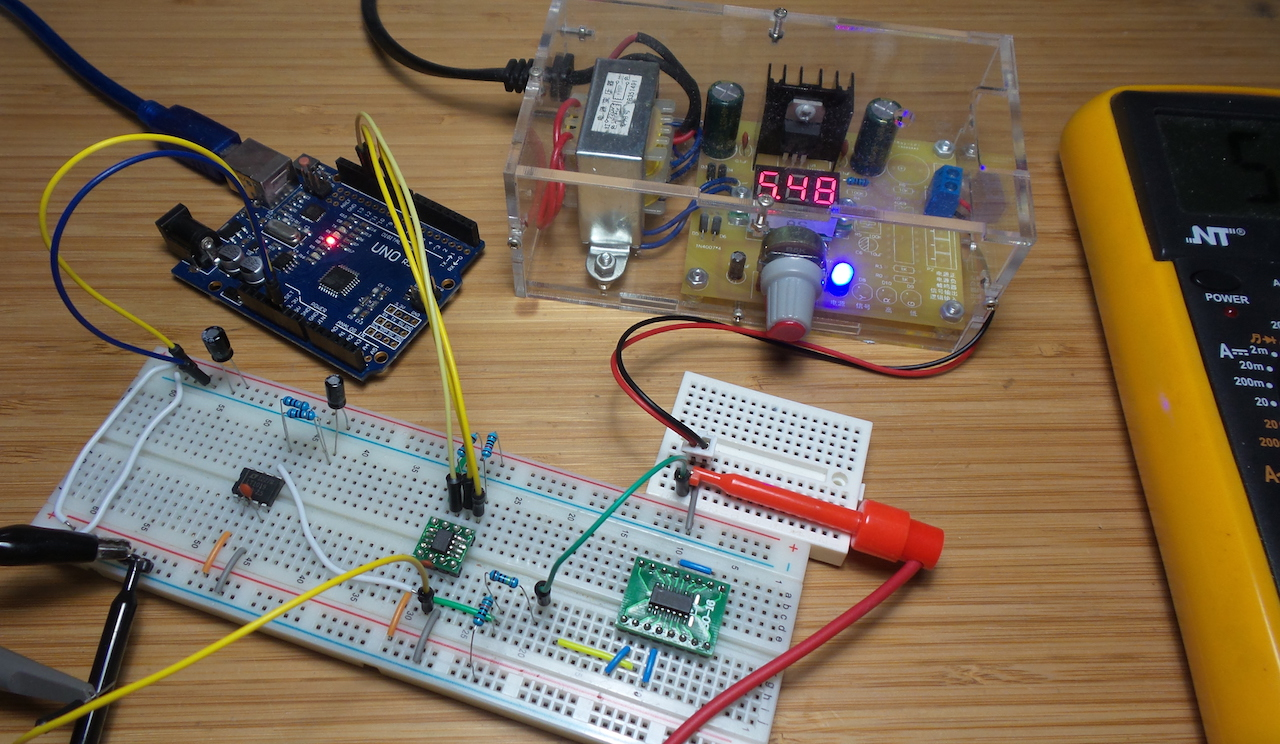I used a combination of an LTC2400 ADC with LT1019 2.5V voltage reference and voltage divider front-end for the LEAP#372 MilliVoltmeter build.

But as @alex1sh pointed out in #11, the accuracy is far from ideal (in my case it skews from +27mV for low voltages to -90mV for high voltages).

The LTC2400 advertises 4ppm full-scale error, so what is going on? That’s what I’m investigating and documenting here.

Note: this is a work-in-progress, as I’ve yet to get good reproduceable results. See the final section of these notes for the current summary and planned next steps.

### Choice Picks from the LTC2400 Datasheet

A close read of the LTC2400 info and datasheet is a good place to start. Some key things I noted:

#### Voltage Reference Range

While Analog Input Voltage is rated for `–0.3V` to `VCC + 0.3V` (p2, Absolute Maximum Ratings), it is qualified with notes, in particular:

Note 14:

• For reference voltage values VREF > 2.5V the extended input of –0.125 • VREF to 1.125 • VREF is limited by the absolute maximum rating of the Analog Input Voltage pin (Pin 3).
• For 2.5V < VREF ≤ 0.267V + 0.89 • VCC the input voltage range is – 0.3V to 1.125 • VREF.
• For 0.267V + 0.89 • VCC < VREF ≤ VCC the input voltage range is – 0.3V to VCC + 0.3V.

Since I am using a 2.5V VREF, and VCC = 5.0V:

With the 1MΩ : 100kΩ voltage divider on the analog input, the maximum input voltage possible before maxing out the reading is therefore 30.94V. At this scaling, 1 bit resolution in the ADC represents 1.77µV input.

#### Output Data Format

Covered on p12, Table 2. LTC2400 Output Data Format:

• input from 0V to VREF, are represented by 0x000000 to 0xFFFFFF in the 24 bits from MSB to LSB of the data frame
• the 4 sub LSBs are valid conversion results beyond the 24-bit level that may be included in averaging or discarded without loss of resolution
• SIG and EXR bits are used to extend the range above VREF and below 0V (subject to limits above)

#### Driving the Input and Reference

From p22..

The LTC2400 is a delta-sigma analog-to-digital converter

The analog input and reference are applied to a switched capacitor network, clocked at 153,600Hz i.e. a 6.5μs sampling period. The switching/sampling actually results in “small” current spikes on VIN and VREF.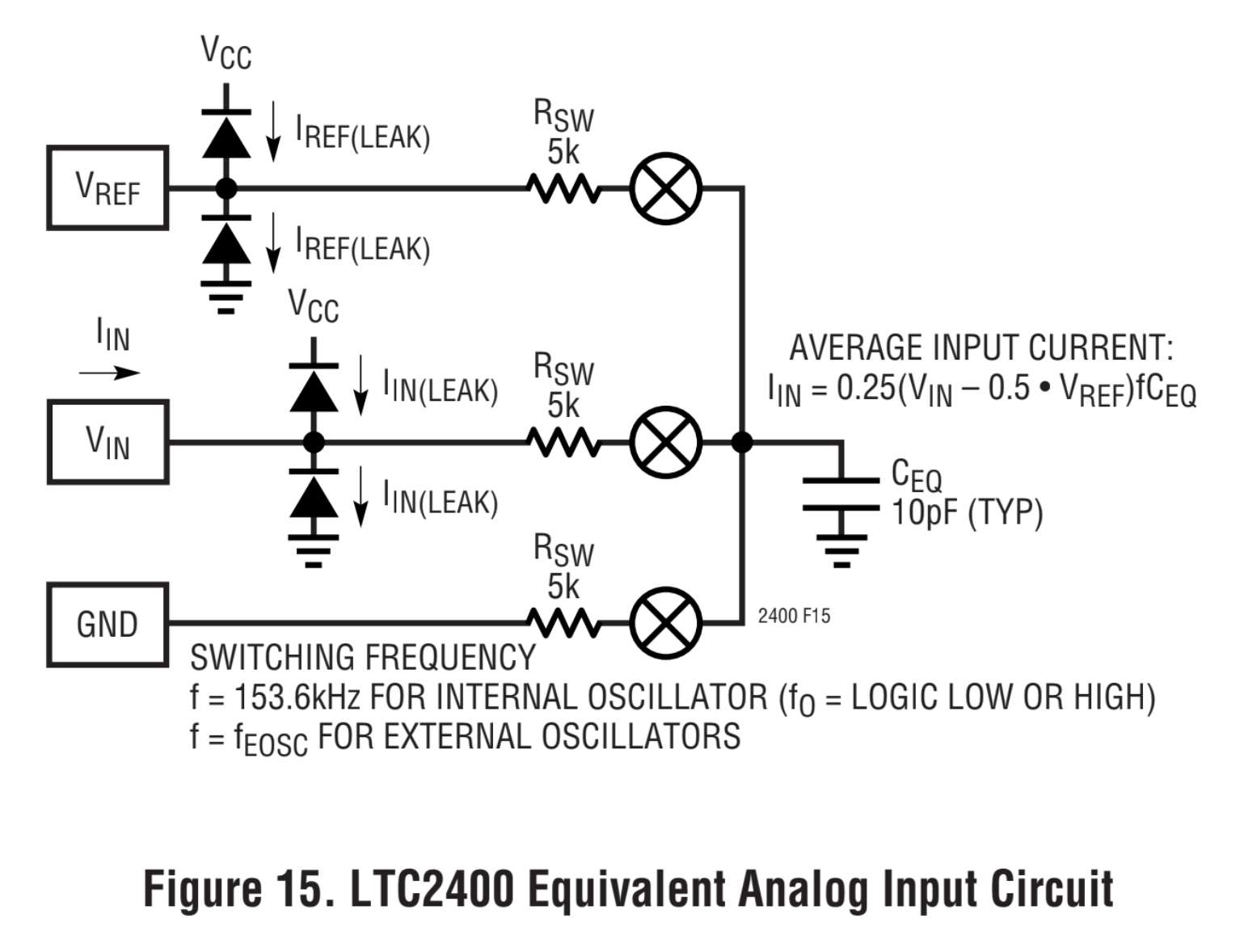This puts an important constraint on the analog input and reference signals:

• the datasheet quotes fourteen time constants required each time a capacitor is switched to achieve 1ppm settling accuracy.
• so time constant at VIN and VREF should be less than 6.5μs/14 = 460ns

Higher resistance and capacitance at VIN contribute to offset and full-scale error. General rule:

• if small capacitance at VIN (<0.01μF), relatively large external source resistances (up to 20k for 20pF parasitic capacitance) can be tolerated without any offset/full-scale error.
• for large input capacitor values (CIN > 0.01μF), the gain shift becomes a linear function of input source resistance independent of input capacitance, corresponding to a 0.3ppm shift in offset and full-scale readings for every 1Ω of input source resistance.

General rule for reference input:

• if capacitance tied to VREF is small (CVREF < 0.01μF), an input resistance of up to 20k (20pF parasitic capacitance at VREF) may be tolerated
• if external capacitance is large (CVREF > 0.01μF)
• full-scale error shift is 0.3ppm/Ω of

#### Application Notes

The LTC2400 info page has a few relevant application notes. Nothing that precisely covers the scenario and issues I’m concerned with, but some points to note:

• most circuits employ a buffer opamp
• few (no?) examples use as external voltage reference. For demonstration purposes, most use VREF = VCC.

## Test Code

In the following scenarios, I’m using the LTC2400.ino sketch to calibrate and perform data logging from the LTC2400. The ADC peripheral interface is implemented in ltc2400_adc.h/ltc2400_adc.cpp.

The sketch is used via the serial interface:

• on start, it asks for the voltage reference to be entered
• performs a calibration to that reference value
• then proceeds to log ADC readings every few seconds. It shows raw ADC values and the implied input voltage based on the calibration factor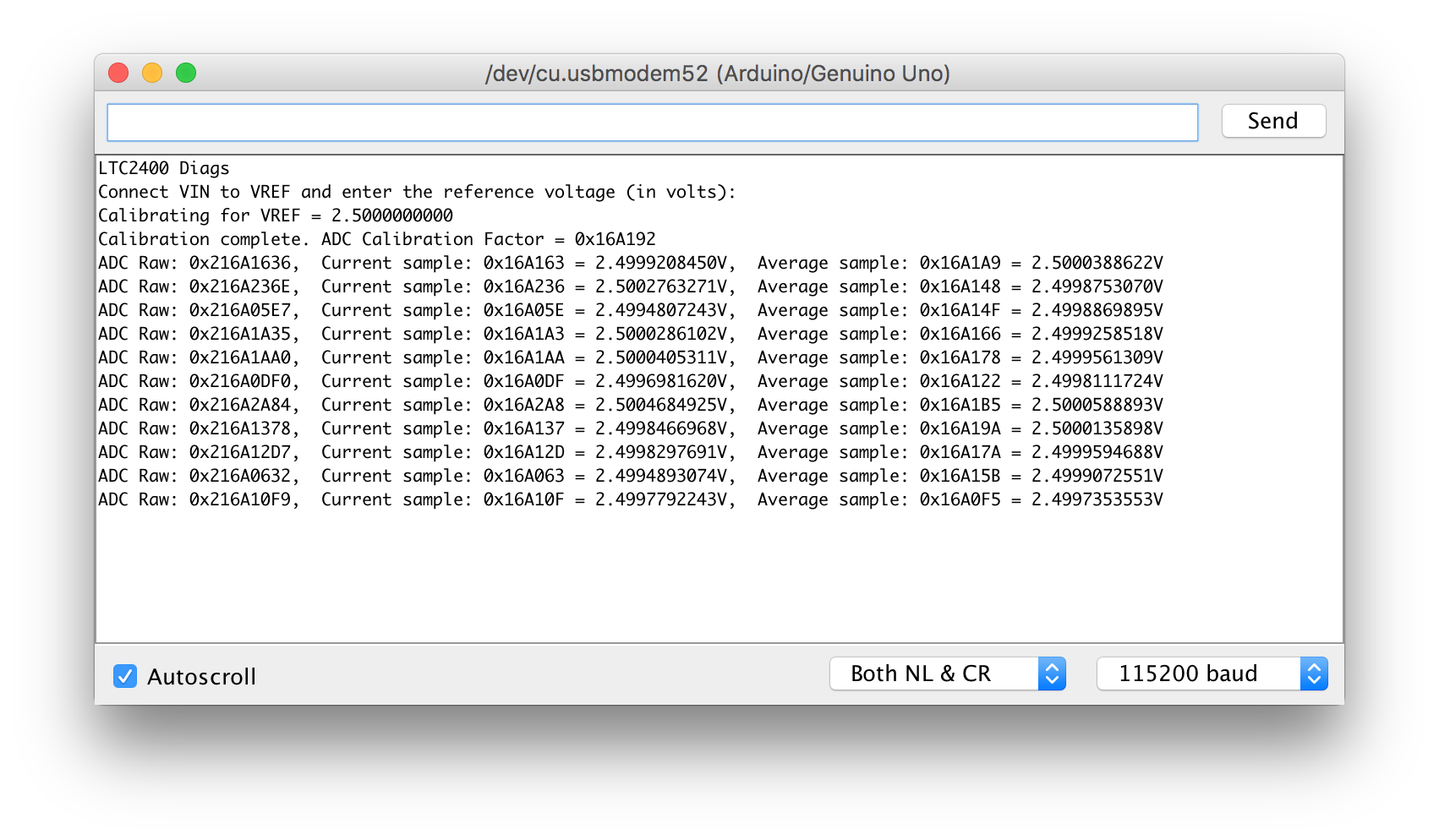## Scenario 1: Baseline Circuit

As a baseline test, I’m first using a reference voltage and ADC circuit identical to the one used for the MilliVoltmeter build.

It is calibrated to 2.5V, and takes an input voltage directly through a 1MΩ:100kΩ voltage divider, without the 10µF C6 installed.

``````LTC2400 Diags
Connect VIN to VREF and enter the reference voltage (in volts):
Calibrating for VREF = 2.5000000000
Calibration complete. ADC Calibration Factor = 0x1745B6
ADC Raw: 0x21745B29,  Current sample: 0x1745B2 = 2.4999933242V,  Average sample: 0x1745C1 = 2.5000181198V
``````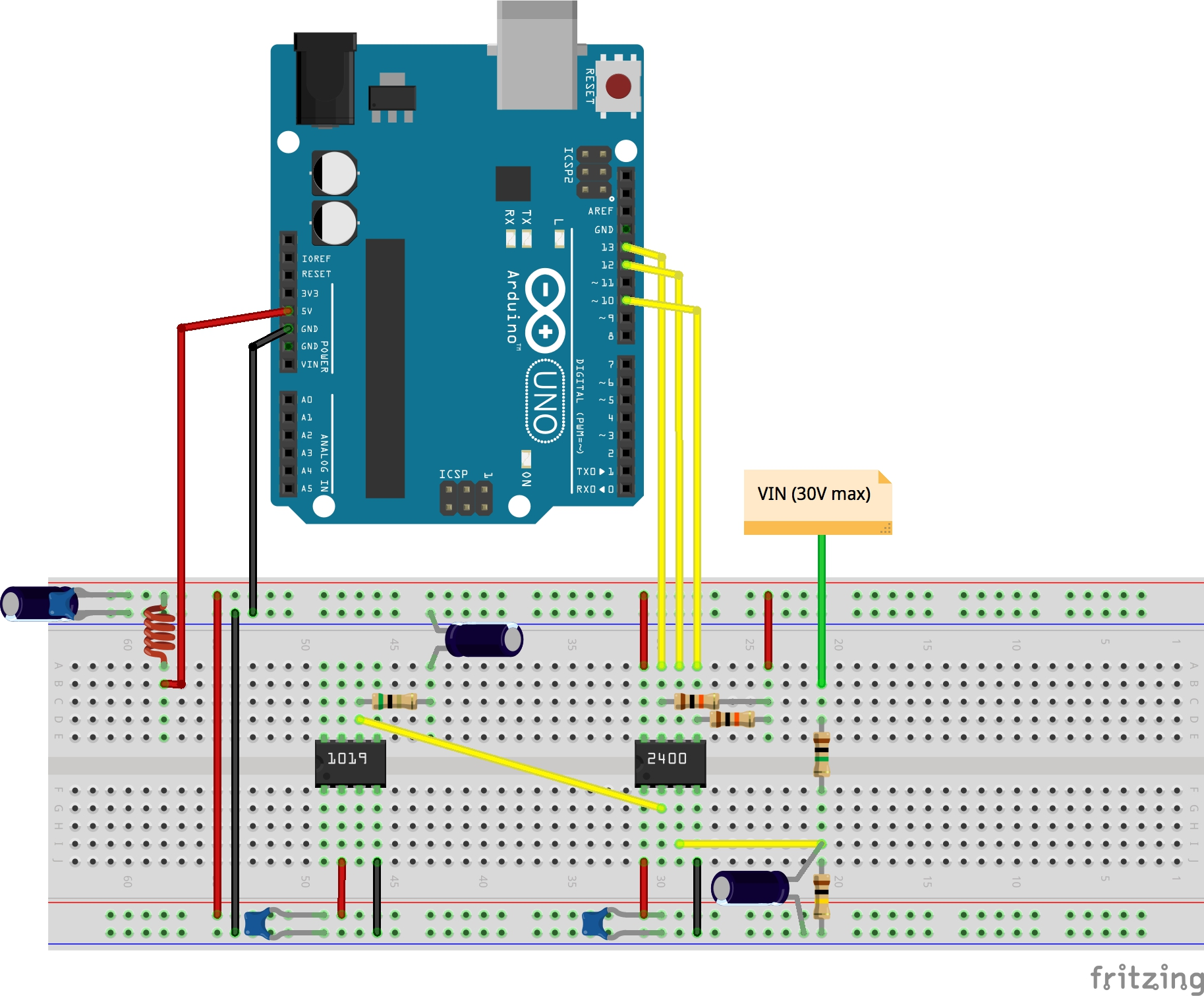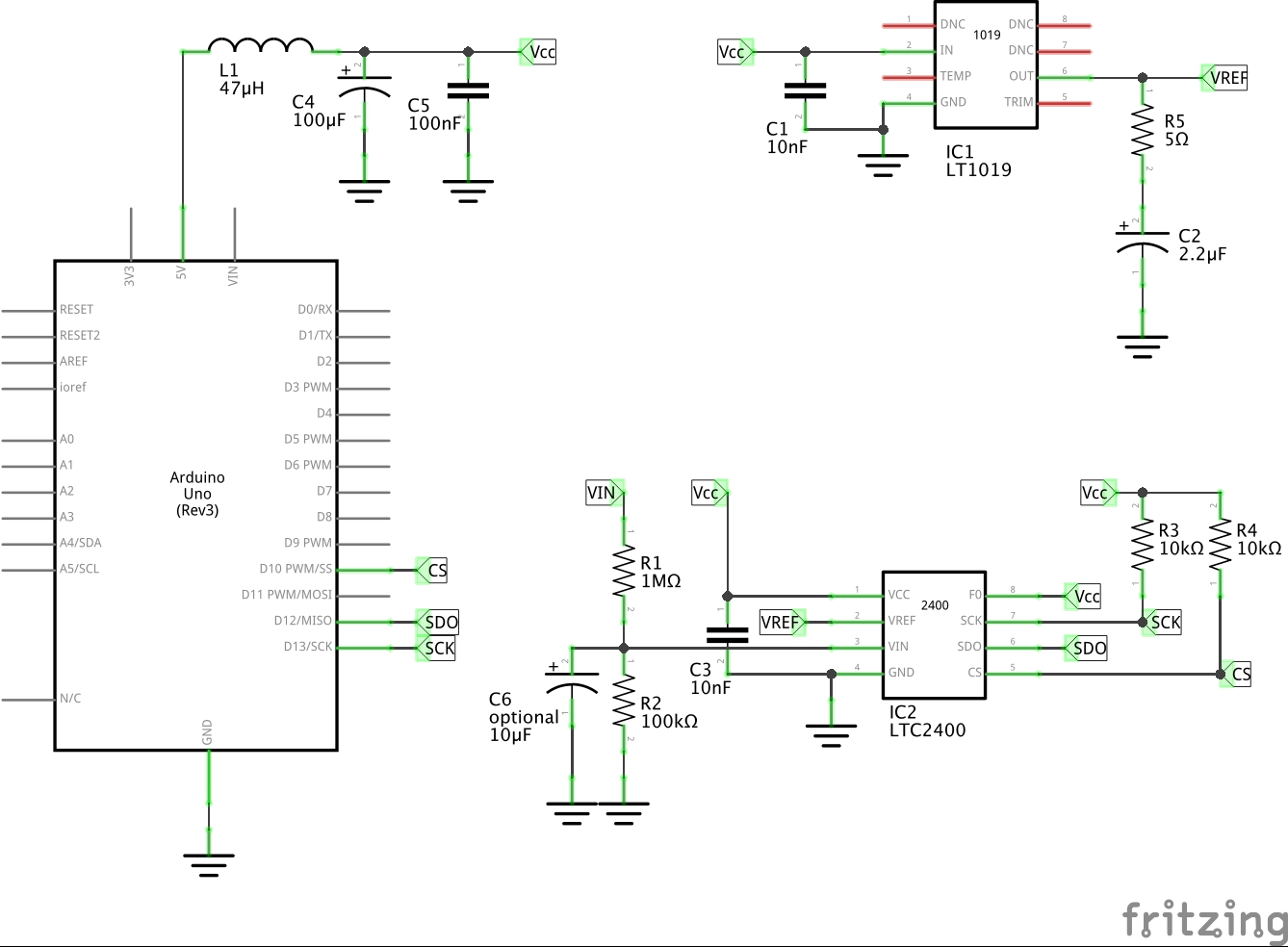1.250 0x20BC961B 0xBC961 1.2661653757V 1.293%
1.901 0x211DC088 0x11DC08 1.9185352325V
5.05 0x22E641F9 0x2E641F 4.9835085868V
7.65 0x24612C32 0x4612C3 7.5275397300V
10.27 0x25DEB659 0x5DEB65 10.0891914367V -1.761%

These readings have significantly skew and error just like I’d seen in the MilliVoltmeter.

Attaching a scope probe to the vin pin of the LTC2400, it is possible to see the effect of the switched-capacitor network during the sampling phase. A series of spikes and discharge curves are present: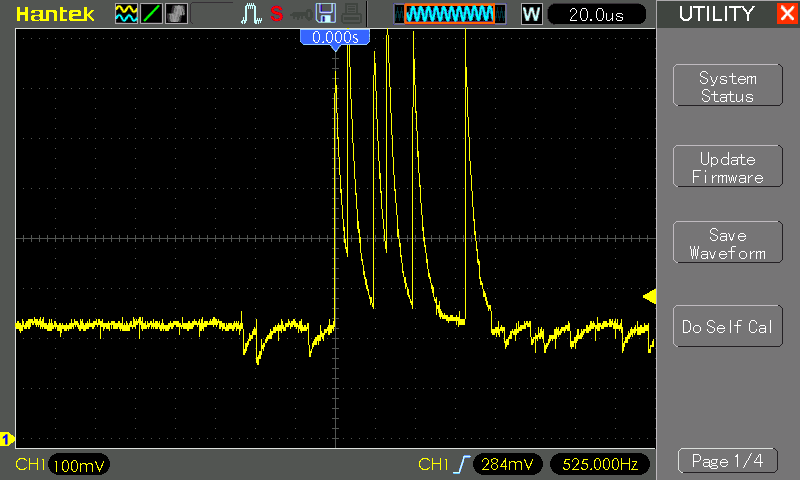## Scenario 2: Capacitor Stabilisation

A quick variation on scenario 1 is to add a 10µF capacitor C6 to stabilise VIN. The 24-Bit A/D Demo Board Schematic from the datasheet (Figure 42) implies this might be a good idea.

But experimentally, when using it to stabilise a 1MΩ:100kΩ voltage divider, the effect is to degrade the accuracy even further.

``````LTC2400 Diags
Connect VIN to VREF and enter the reference voltage (in volts):
Calibrating for VREF = 2.5000000000
Calibration complete. ADC Calibration Factor = 0x17B47C
ADC Raw: 0x21873FC4,  Current sample: 0x1873FC = 2.5788912773V,  Average sample: 0x17D9B6 = 2.5153360366V
``````
1.253 0x20B3D923 0xB3D92 1.1854567527V -5.39%
10.18 0x25DCF857 0x5DCF85 9.8935403823V -2.814%

As might be expected, the additional large-value capacitor is significantly affecting the switched-capacitor network during the sampling phase: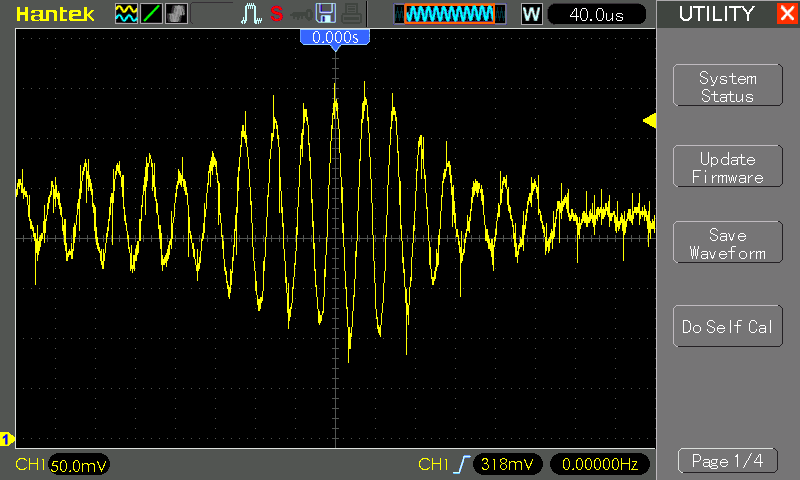## Scenario 3: Buffered VREF and VIN Signals

Given the non-trivial impact that the switched-capacitor network of the LTC2400 has on reference and input signals, it follows that buffering these inputs may lead to better stability and accuracy.

This technique is actually described in many of the examples in application note AN78-8 “A Collection of Differential to Single-Ended Signal Conditioning Circuits for Use with the LTC2400”.

For this test, I have an LMV324 buffering the 2.5V voltage reference in two independent buffers:

• one buffer feeds the LTC2400 reference input
• the other buffer is directly feeding the LTC2400 vin

In this configuration, once would expect:

• the ADC reading should be 0xFFFFFF, i.e. exactly equal to the reference voltage
• errors and fluctuations should be minimised

Here’s an example trace:

``````LTC2400 Diags
Connect VIN to VREF and enter the reference voltage (in volts):
Calibrating for VREF = 2.5000000000
Calibration complete. ADC Calibration Factor = 0xFFEF28
ADC Raw: 0x2FFEEDB9,  Current sample: 0xFFEEDB = 2.4999885559V,  Average sample: 0xFFEED5 = 2.4999876022V
ADC Raw: 0x2FFF18C1,  Current sample: 0xFFF18C = 2.5000913143V,  Average sample: 0xFFEF24 = 2.4999992847V
ADC Raw: 0x2FFF0795,  Current sample: 0xFFF079 = 2.5000503063V,  Average sample: 0xFFEF43 = 2.5000040531V
ADC Raw: 0x2FFF1676,  Current sample: 0xFFF167 = 2.5000855922V,  Average sample: 0xFFEF8E = 2.5000152587V
ADC Raw: 0x2FFF1DC1,  Current sample: 0xFFF1DC = 2.5001032352V,  Average sample: 0xFFEFC0 = 2.5000226497V
ADC Raw: 0x2FFF1F80,  Current sample: 0xFFF1F8 = 2.5001072883V,  Average sample: 0xFFF01B = 2.5000362396V
ADC Raw: 0x2FFF1B08,  Current sample: 0xFFF1B0 = 2.5000965595V,  Average sample: 0xFFF069 = 2.5000479221V
ADC Raw: 0x2FFF1AF7,  Current sample: 0xFFF1AF = 2.5000963211V,  Average sample: 0xFFF0BC = 2.5000603199V
ADC Raw: 0x2FFF1DA8,  Current sample: 0xFFF1DA = 2.5001027584V,  Average sample: 0xFFF106 = 2.5000712871V
ADC Raw: 0x2FFF20D1,  Current sample: 0xFFF20D = 2.5001103878V,  Average sample: 0xFFF156 = 2.5000832080V
ADC Raw: 0x2FFF207B,  Current sample: 0xFFF207 = 2.5001094341V,  Average sample: 0xFFF1A7 = 2.5000951290V
ADC Raw: 0x2FFF1F1D,  Current sample: 0xFFF1F1 = 2.5001063346V,  Average sample: 0xFFF1B1 = 2.5000967979V
ADC Raw: 0x2FFF22AB,  Current sample: 0xFFF22A = 2.5001146793V,  Average sample: 0xFFF1DD = 2.5001032352V
ADC Raw: 0x2FFF20CA,  Current sample: 0xFFF20C = 2.5001103878V,  Average sample: 0xFFF1ED = 2.5001056194V
ADC Raw: 0x2FFF2A45,  Current sample: 0xFFF2A4 = 2.5001327991V,  Average sample: 0xFFF201 = 2.5001087188V
ADC Raw: 0x2FFF209B,  Current sample: 0xFFF209 = 2.5001099109V,  Average sample: 0xFFF203 = 2.5001089572V
ADC Raw: 0x2FFF21C6,  Current sample: 0xFFF21C = 2.5001127719V,  Average sample: 0xFFF20E = 2.5001106262V
ADC Raw: 0x2FFF1C5C,  Current sample: 0xFFF1C5 = 2.5000996589V,  Average sample: 0xFFF210 = 2.5001108646V
``````

That is a disappointing result on two points:

• the average ADC reading 0xFFF18A is offset from the expected 0xFFFFFF by -0.022%
• and the results vary by a range of 0x3C9. Given that readings are in the order of 16 million, that represents a range of around 60 ppm - far above the stated error bands in the datasheet.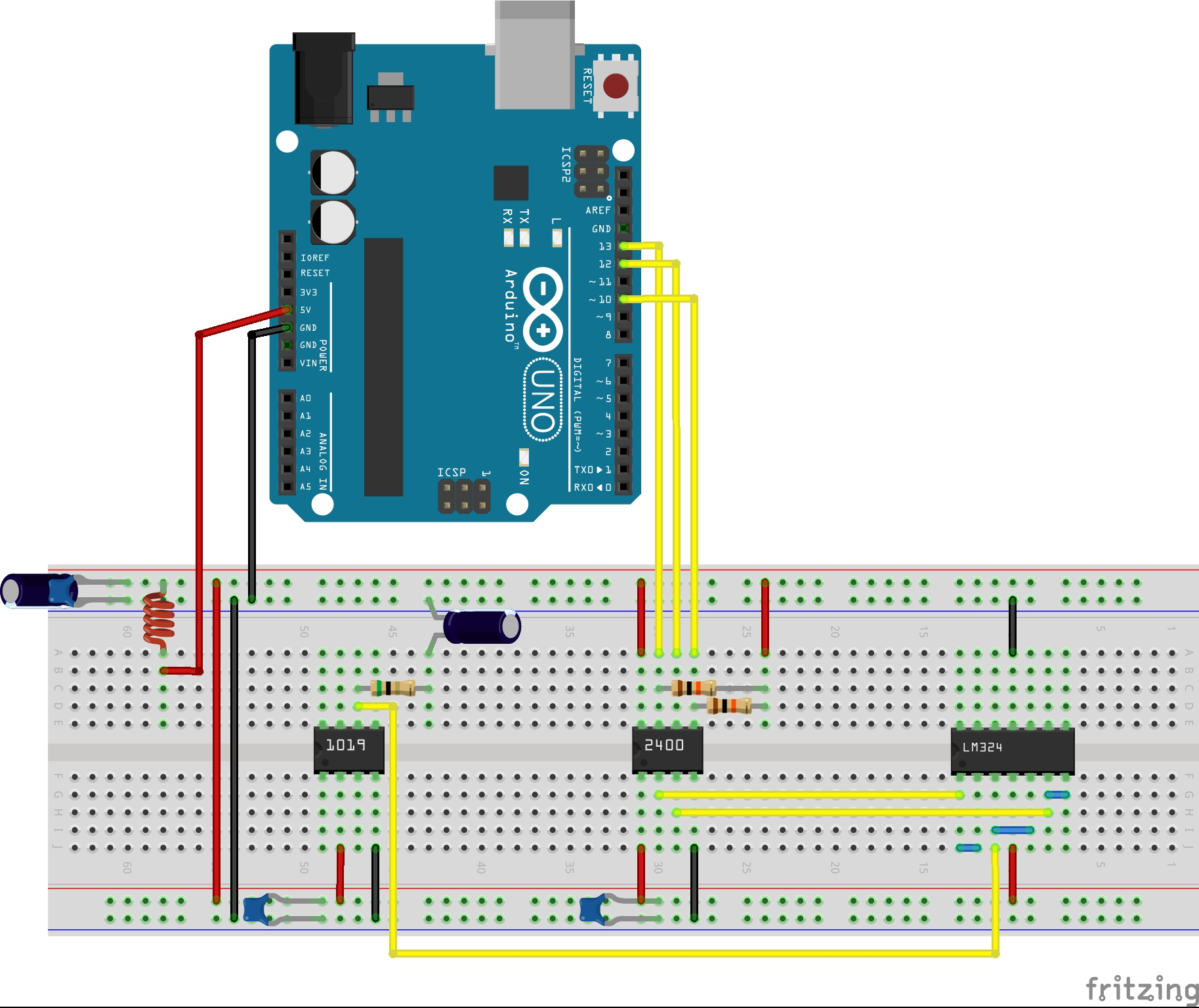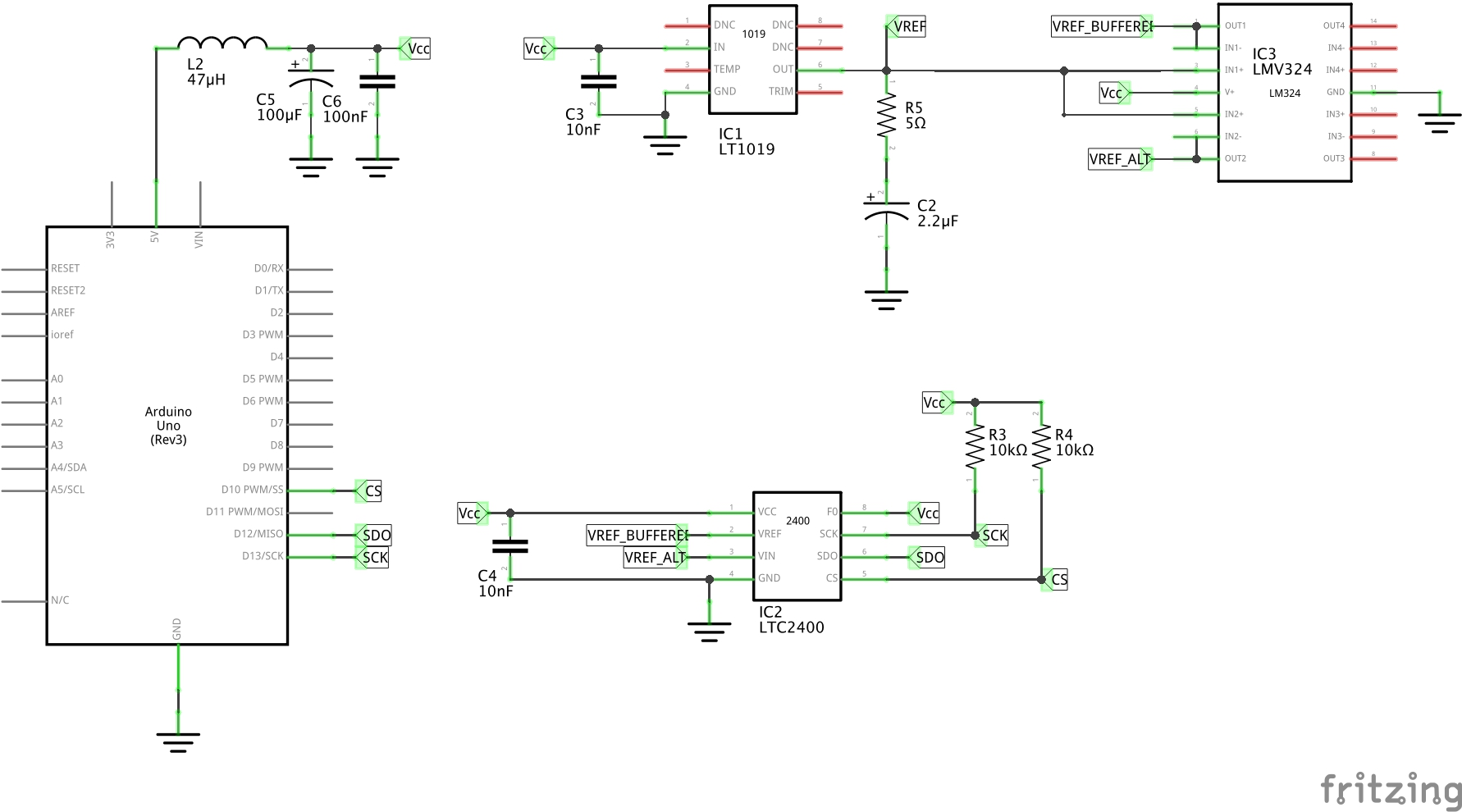## Regrouping / Conclusions to-date

My next steps would be to test buffered input with and without a voltage divider, however the error encountered in Scenario 3: Buffered VREF and VIN Signals give some pause: if acceptable error levels cannot be maintained in this case, it would be reasonable to expect the further tests would fair no better, and likely perform worse.

So what could be contributing to the error levels I’m seeing. Some possibilities:

1. excessive noise in the experimental setup. All the tests so far have been performed on a breadboard, using the 5V supply form the Arduino
2. the LMV324 op-amp buffer varies from the ideal buffer model and actively interferes with the switched-capacitor sampling model used by the LTC2400
3. faulty components? The LT1019 and LTC2400 chips I’m using ware obtained from a reputable channel so I would not blame the parts in the first instance. I have also switched chips along the way and results remain consistent
4. I’ve made some fundamental mistake here in the application, or understanding of how to correctly use the LTC2400

I would guess that (1) and (2) are the most likely problem areas, and also the ones easiest to test further. I think my next steps may be to:

1. Put the circuit on a PCB, perhaps Manhattan style over a solid ground plane and an independent low-noise power supply
2. Replace the LMV324 op-amp with either:
• LT1077 precision op-amp, as the LT1077 is the part used in LTC2400 reference designs in application notes so may be assumed to be “well matched”. The LT1077 has much better voltage offset and drift specifications and also a lower slew rate which may be beneficial in this application
• another alternative is the OP07DD, as it’s also been seen in precision analog front-end designs and better specs than the LMV324
• or the AD8628ARZ as used in the Scullcom millivoltmeter design and better specs than the LMV324

Comparing op-amp options:

Measure LMV324 LT1077 OP07DD AD8628ARZ OPA2388
Max Supply Current 200µA 60µA
Max Offset Voltage 3mV 40µV 150µV 5µV ±5µV
Max Offset Current 9nA 350pA 6nA 200pA ±700pA
Voltage Noise ? 0.5µVp-p 0.1Hz to 10Hz   0.5µVp-p 0.1Hz to 10Hz 0.14µVp-p
Current Noise ? 2.5pAp-p 0.1Hz to 10Hz
Offset Voltage Drift 2µV/°C 0.4µV/°C 2.5µV/°C 0.02µV/°C ±0.05µV/°C
Gain-Bandwidth Product 1MHz 250kHz 0.6MHz 2.5MHz 10MHz
Slew Rate 0.35V/µs 0.12V/µs 0.3V/µs 1V/µs 5V/µs

## Scenario 4: OPA2388-buffered VREF Signals

I got hold of some precision opamps to see if they can improve matters. For a first test I’ll use an OPA2388 to buffer VREF, and read a 1:1 buffered VREF in at the ADC.

This should produce the most reliable reading possible. In this configuration, VREF is buffered twice:

• one op-amp provides a buffered reference voltage for the LTC2400
• the other op-amp provides the input voltage for the LTC2400

In this configuration, once would expect:

• the ADC reading should be 0xFFFFFF, i.e. exactly equal to the reference voltage
• errors and fluctuations should be minimised

Here’s an example trace:

``````LTC2400 Diags
Connect VIN to VREF and enter the reference voltage (in volts):
Calibrating for VREF = 2.5000000000
Calibration complete. ADC Calibration Factor = 0x1000059
ADC Raw: 0x300005C7,  Current sample: 0x100005C = 2.5000007152V,  Average sample: 0x1000059 = 2.5000000000V
ADC Raw: 0x300005FA,  Current sample: 0x100005F = 2.5000011920V,  Average sample: 0x100005C = 2.5000007152V
ADC Raw: 0x300005A0,  Current sample: 0x100005A = 2.5000002384V,  Average sample: 0x100005D = 2.5000007152V
ADC Raw: 0x3000073E,  Current sample: 0x1000073 = 2.5000040531V,  Average sample: 0x1000062 = 2.5000014305V
ADC Raw: 0x30000471,  Current sample: 0x1000047 = 2.4999976158V,  Average sample: 0x100005E = 2.5000009536V
ADC Raw: 0x3000075A,  Current sample: 0x1000075 = 2.5000040531V,  Average sample: 0x1000060 = 2.5000011920V
ADC Raw: 0x30000452,  Current sample: 0x1000045 = 2.4999969005V,  Average sample: 0x100005D = 2.5000007152V
ADC Raw: 0x300005EB,  Current sample: 0x100005E = 2.5000009536V,  Average sample: 0x100005C = 2.5000007152V
ADC Raw: 0x3000064F,  Current sample: 0x1000064 = 2.5000016689V,  Average sample: 0x100005B = 2.5000007152V
ADC Raw: 0x30000702,  Current sample: 0x1000070 = 2.5000035762V,  Average sample: 0x100005F = 2.5000011920V
ADC Raw: 0x3000078A,  Current sample: 0x1000078 = 2.5000047683V,  Average sample: 0x1000062 = 2.5000014305V
ADC Raw: 0x30000531,  Current sample: 0x1000053 = 2.4999992847V,  Average sample: 0x1000061 = 2.5000011920V
ADC Raw: 0x3000071D,  Current sample: 0x1000071 = 2.5000035762V,  Average sample: 0x1000063 = 2.5000016689V
ADC Raw: 0x3000059B,  Current sample: 0x1000059 = 2.5000000000V,  Average sample: 0x1000060 = 2.5000011920V
ADC Raw: 0x3000064A,  Current sample: 0x1000064 = 2.5000016689V,  Average sample: 0x1000063 = 2.5000016689V
ADC Raw: 0x300006C3,  Current sample: 0x100006C = 2.5000030994V,  Average sample: 0x1000062 = 2.5000014305V
``````

That is a much more encouraging result:

• the average result reading is 0x1000062 (2.50000151V), an error of only 0.00006%
• and the results vary by a range of 0x33. Given that readings are in the order of 16 million, that represents a range of around 3 ppm - just under the 4ppm full-scale error reported in the datasheet.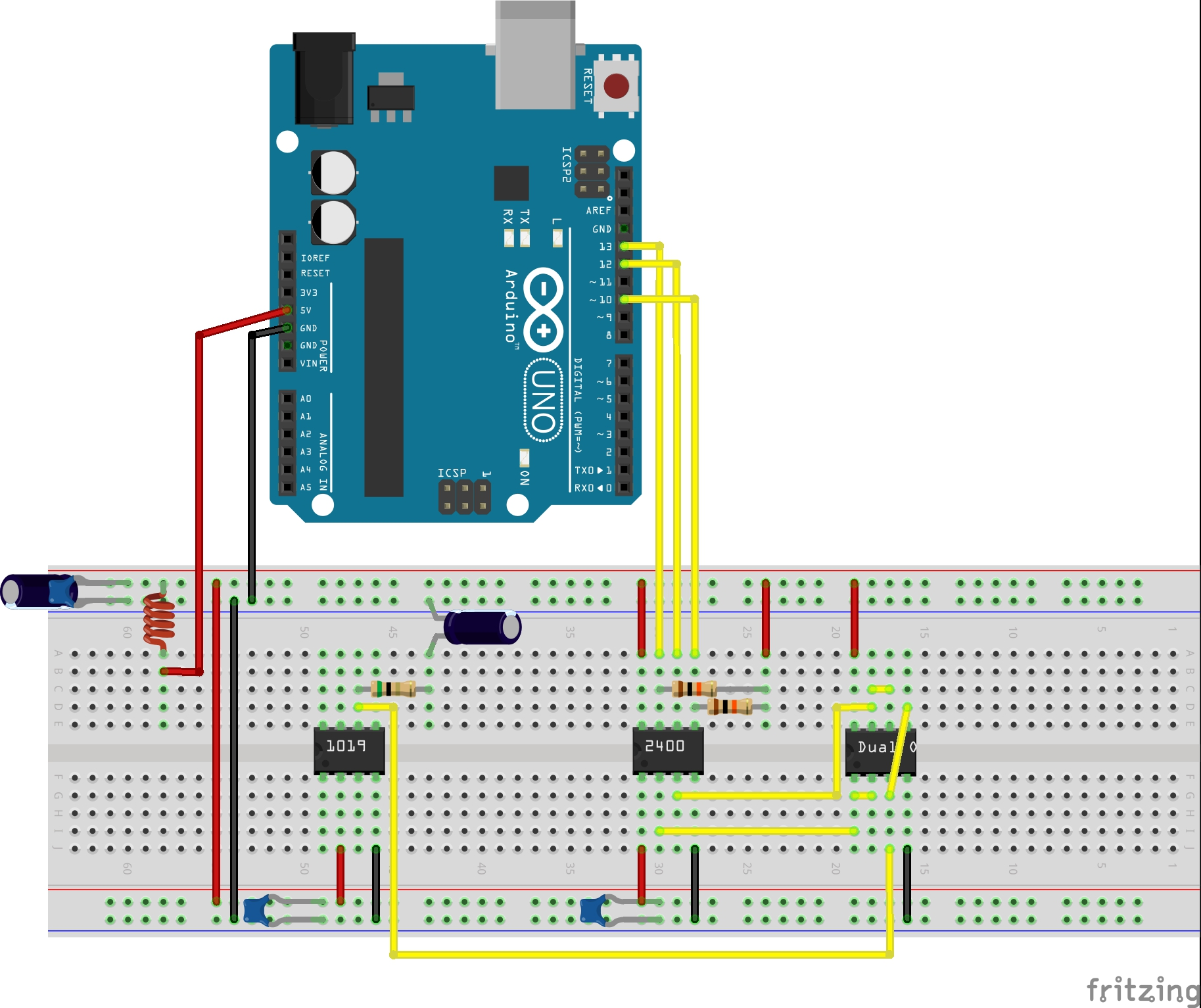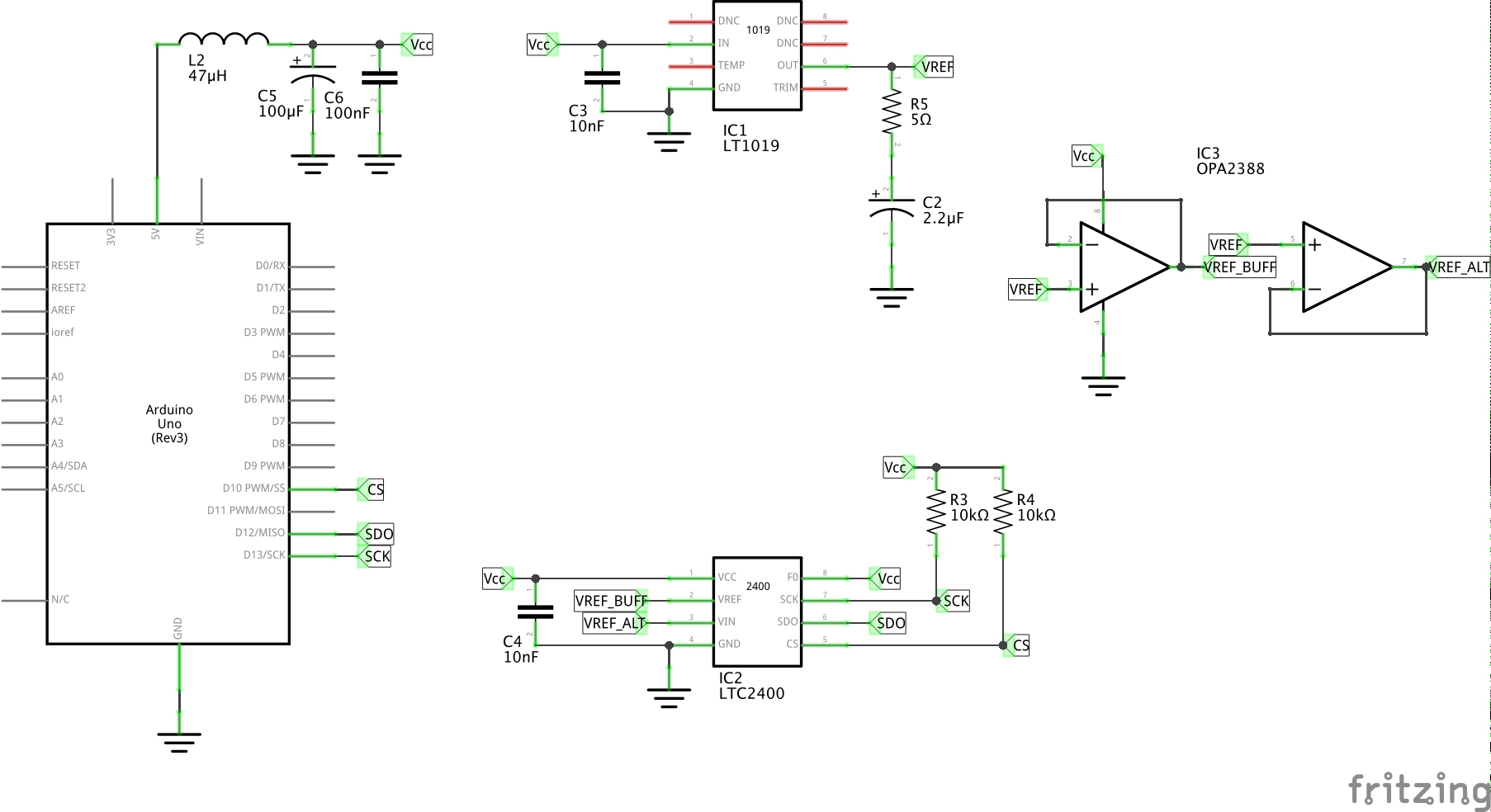## Scenario 5: OPA2388-buffered Everything

This variant of the circuit re-introduces the 1MΩ:100kΩ voltage divider on the input. Three OPAx388 buffers are used to prevent coupling of signals:

• VREF buffered between LT1019 and LTC2400
• VREF buffered to act as independant voltage reference
• VIN buffered from the voltage divider

The independant 2.5V voltage reference is used to feed VIN via the voltage reference, the idea being that we should be able to read a clean and accurate 2.5V signal via the voltage reference.

A set of sample results:

``````LTC2400 Diags
Connect VIN to VREF and enter the reference voltage (in volts):
Calibrating for VREF = 2.5000000000
Calibration complete. ADC Calibration Factor = 0x16C6A6
ADC Raw: 0x216C7B33,  Current sample: 0x16C7B3 = 2.5004506111V,  Average sample: 0x16C6A8 = 2.5000033378V
ADC Raw: 0x216C807D,  Current sample: 0x16C807 = 2.5005912780V,  Average sample: 0x16C6D5 = 2.5000786781V
ADC Raw: 0x216C4F83,  Current sample: 0x16C4F8 = 2.4992797374V,  Average sample: 0x16C6A5 = 2.4999983310V
ADC Raw: 0x216C671A,  Current sample: 0x16C671 = 2.4999113082V,  Average sample: 0x16C6B3 = 2.5000216960V
ADC Raw: 0x216C56AD,  Current sample: 0x16C56A = 2.4994707107V,  Average sample: 0x16C692 = 2.4999666213V
ADC Raw: 0x216C6502,  Current sample: 0x16C650 = 2.4998559951V,  Average sample: 0x16C687 = 2.4999480247V
ADC Raw: 0x216C6D1A,  Current sample: 0x16C6D1 = 2.5000720024V,  Average sample: 0x16C684 = 2.4999430179V
ADC Raw: 0x216C6F49,  Current sample: 0x16C6F4 = 2.5001306533V,  Average sample: 0x16C697 = 2.4999749660V
ADC Raw: 0x216C6B17,  Current sample: 0x16C6B1 = 2.5000183582V,  Average sample: 0x16C6A6 = 2.5000000000V
ADC Raw: 0x216C579A,  Current sample: 0x16C579 = 2.4994957447V,  Average sample: 0x16C67A = 2.4999263286V
ADC Raw: 0x216C5202,  Current sample: 0x16C520 = 2.4993467330V,  Average sample: 0x16C638 = 2.4998157024V
ADC Raw: 0x216C483F,  Current sample: 0x16C483 = 2.4990837574V,  Average sample: 0x16C5DE = 2.4996650218V
ADC Raw: 0x216C4536,  Current sample: 0x16C453 = 2.4990034103V,  Average sample: 0x16C5CE = 2.4996383190V
ADC Raw: 0x216C5DF7,  Current sample: 0x16C5DF = 2.4996666908V,  Average sample: 0x16C5BF = 2.4996130466V
ADC Raw: 0x216C4E35,  Current sample: 0x16C4E3 = 2.4992446899V,  Average sample: 0x16C5B2 = 2.4995913505V
ADC Raw: 0x216C4AA0,  Current sample: 0x16C4AA = 2.4991490840V,  Average sample: 0x16C588 = 2.4995210170V
ADC Raw: 0x216C4E82,  Current sample: 0x16C4E8 = 2.4992530345V,  Average sample: 0x16C557 = 2.4994390010V
ADC Raw: 0x216C3A5E,  Current sample: 0x16C3A5 = 2.4987120628V,  Average sample: 0x16C502 = 2.4992966651V
ADC Raw: 0x216C5A92,  Current sample: 0x16C5A9 = 2.4995763301V,  Average sample: 0x16C4E8 = 2.4992530345V
ADC Raw: 0x216C5A9F,  Current sample: 0x16C5A9 = 2.4995763301V,  Average sample: 0x16C4EC = 2.4992597103V
ADC Raw: 0x216C5EAE,  Current sample: 0x16C5EA = 2.4996850490V,  Average sample: 0x16C501 = 2.4992947578V
ADC Raw: 0x216C6E48,  Current sample: 0x16C6E4 = 2.5001039505V,  Average sample: 0x16C53E = 2.4993970394V
ADC Raw: 0x216C56EE,  Current sample: 0x16C56E = 2.4994773864V,  Average sample: 0x16C55A = 2.4994440078V
ADC Raw: 0x216C5A49,  Current sample: 0x16C5A4 = 2.4995679855V,  Average sample: 0x16C554 = 2.4994339942V
ADC Raw: 0x216C6D3D,  Current sample: 0x16C6D3 = 2.5000753402V,  Average sample: 0x16C586 = 2.4995176792V
ADC Raw: 0x216C5B9B,  Current sample: 0x16C5B9 = 2.4996030330V,  Average sample: 0x16C5A1 = 2.4995627403V
ADC Raw: 0x216C4E4D,  Current sample: 0x16C4E4 = 2.4992463588V,  Average sample: 0x16C5A0 = 2.4995610713V
ADC Raw: 0x216C4793,  Current sample: 0x16C479 = 2.4990670680V,  Average sample: 0x16C5B5 = 2.4995963573V
ADC Raw: 0x216C67BC,  Current sample: 0x16C67B = 2.4999279975V,  Average sample: 0x16C5CA = 2.4996316432V
ADC Raw: 0x216C3626,  Current sample: 0x16C362 = 2.4985997676V,  Average sample: 0x16C590 = 2.4995343685V
ADC Raw: 0x216C4FEA,  Current sample: 0x16C4FE = 2.4992897510V,  Average sample: 0x16C579 = 2.4994957447V
ADC Raw: 0x216C57FF,  Current sample: 0x16C57F = 2.4995059967V,  Average sample: 0x16C555 = 2.4994356632V
``````

These results are not bad with 0.08% variability. Results are summarised below, along with some simple variations:

• Base: the circuit as described
• SuperBypass: with extra 100nF and 100µF bypass caps on the OPA2388 and LTC2400
• DedicatedPower: with separate 5V power supply, insteda of using the 5V from the Arduino.
Base SuperBypass DedicatedPower
average 2.499574749 2.499558449 2.499595125
min 2.498599768 2.498459816 2.498825788
max 2.500591278 2.500326395 2.500210762
range 0.0019915104 0.0018665791 0.0013849736
range % 0.08% 0.07% 0.06%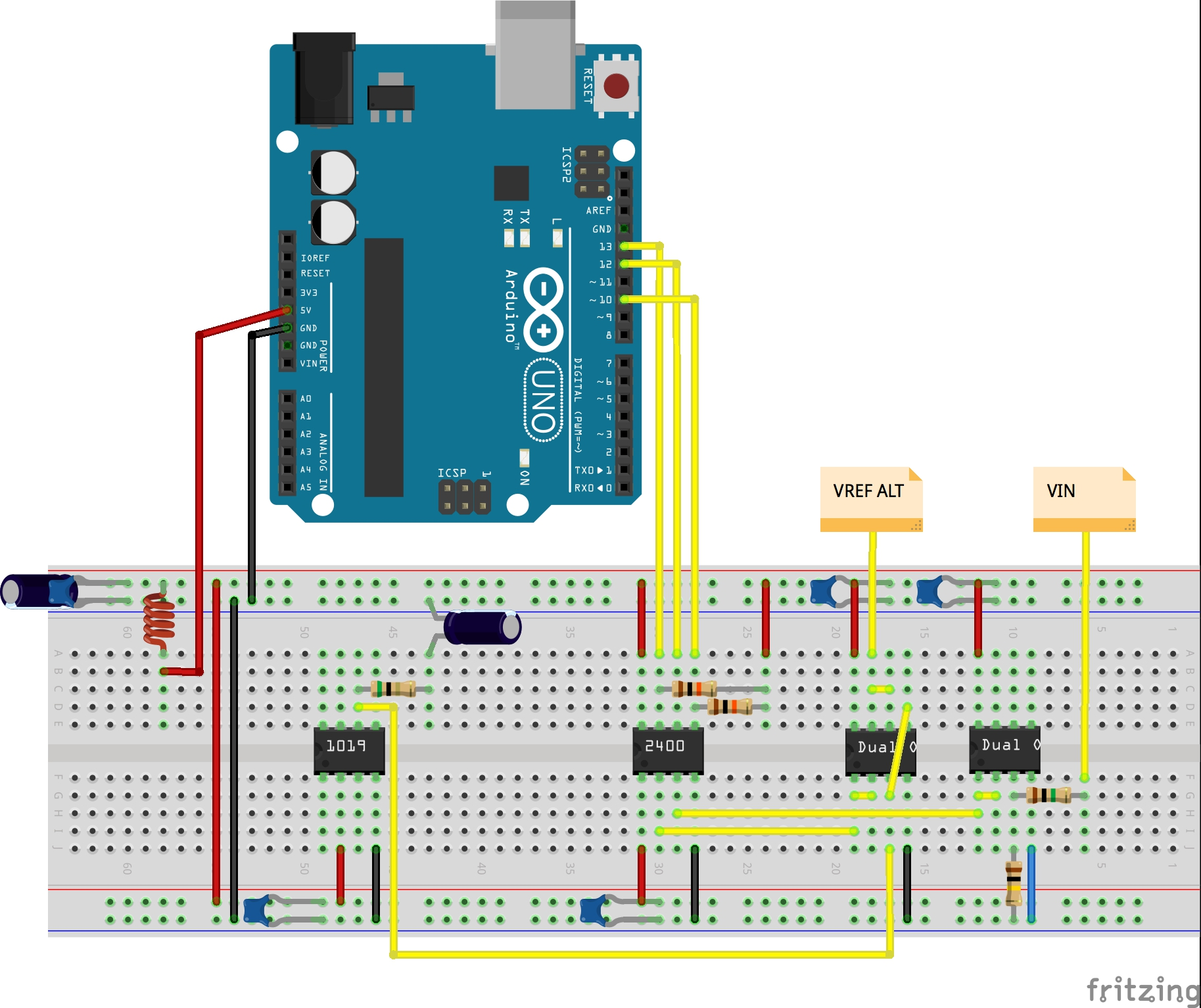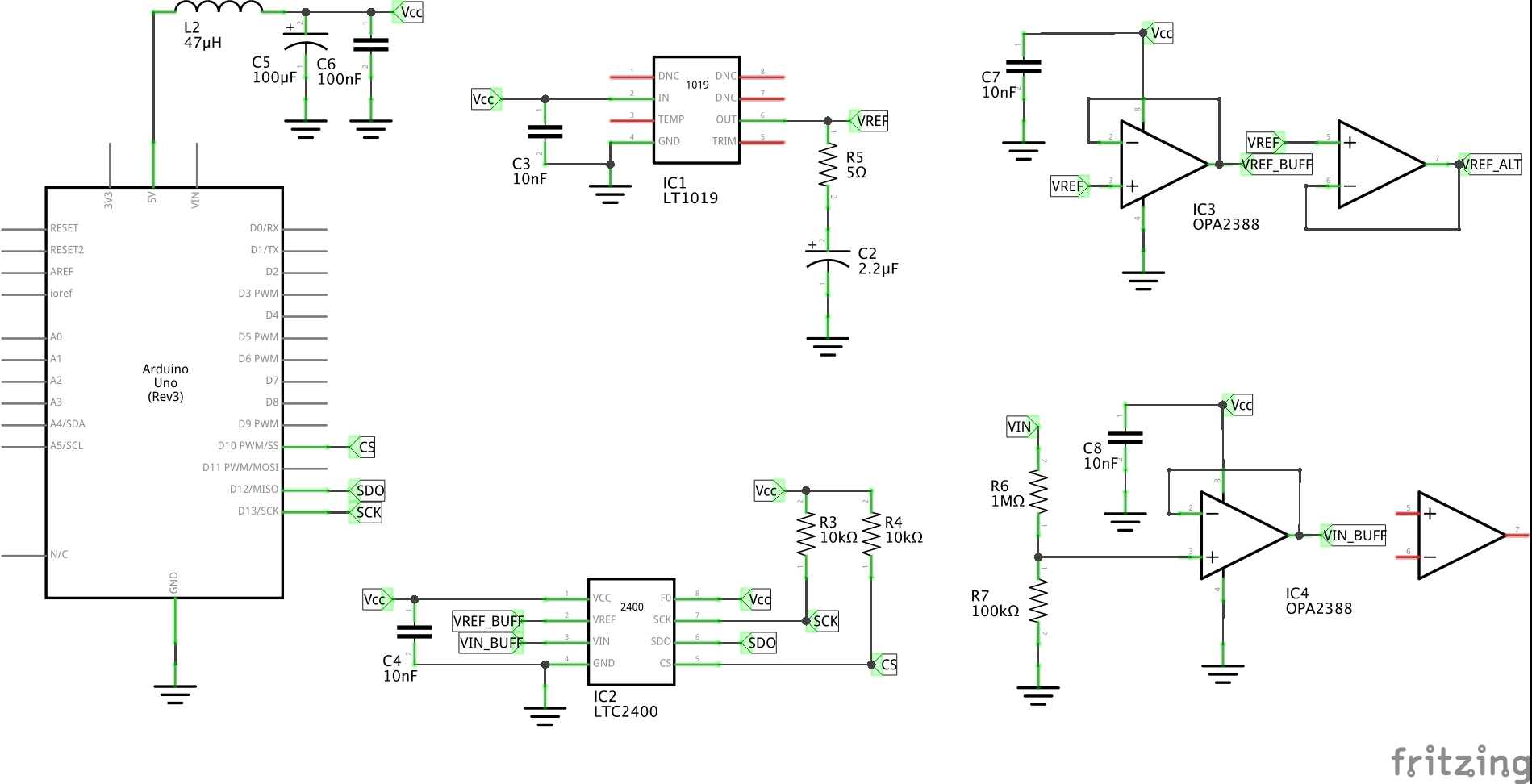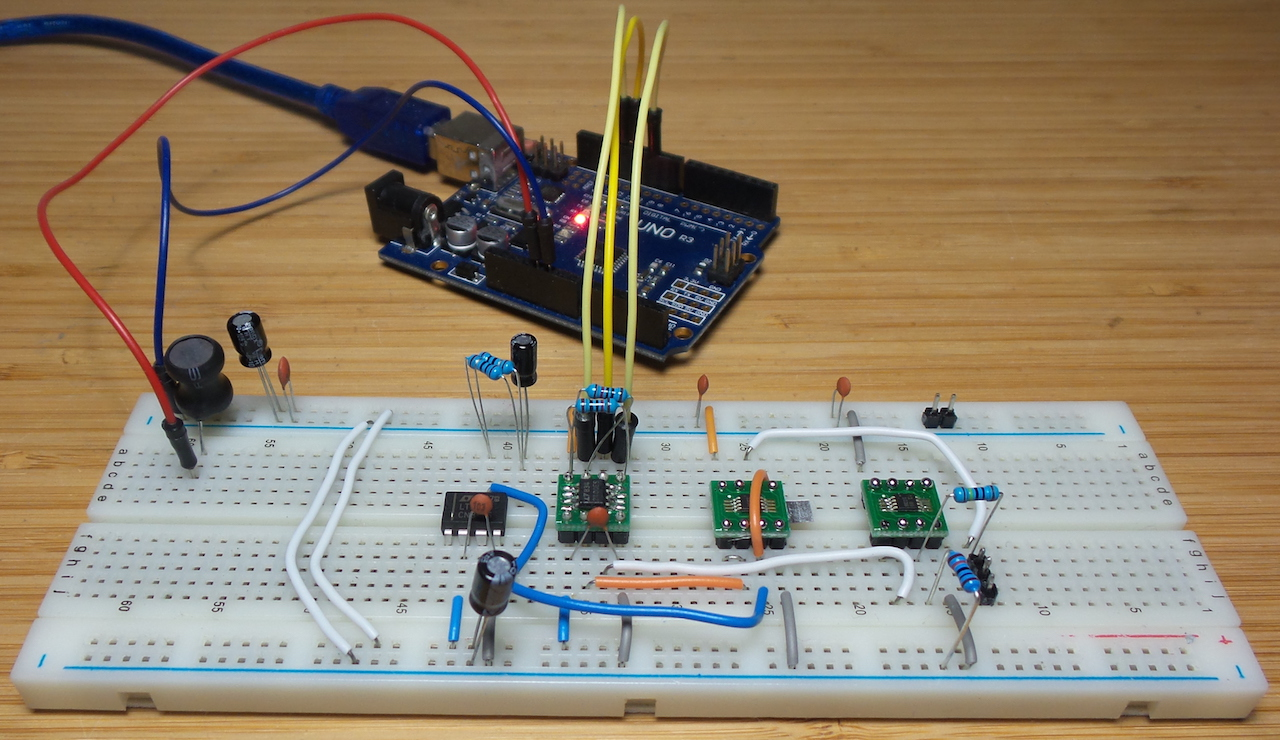## Scenario 6: ADR4540 Reference with OPA2388-buffered Everything

This variant switches out the 2.5V LT1019 voltage reference for a 4.096V ADR4540 reference. The higher reference may improve stability and accuracy by increasing the dynamic range of VIN.

As with Scenario 5, the circuit use the 1MΩ:100kΩ voltage divider on the input. Three OPAx388 buffers are used to prevent coupling of signals:

• VREF buffered between ADR4540 and LTC2400
• VREF buffered to act as independant voltage reference
• VIN buffered from the voltage divider

The independant 4.096V voltage reference is used to feed VIN via the voltage reference, the idea being that we should be able to read a clean and accurate 4.096V signal via the voltage reference.

A set of sample results:

``````LTC2400 Diags
Connect VIN to VREF and enter the reference voltage (in volts):
Calibrating for VREF = 4.0960001945
Calibration complete. ADC Calibration Factor = 0x16CF1C
ADC Raw: 0x216CF4D1,  Current sample: 0x16CF4D = 4.0961346626V,  Average sample: 0x16CF0A = 4.0959510803V
ADC Raw: 0x216CF072,  Current sample: 0x16CF07 = 4.0959424972V,  Average sample: 0x16CF08 = 4.0959453582V
ADC Raw: 0x216CEA38,  Current sample: 0x16CEA3 = 4.0956683158V,  Average sample: 0x16CEF1 = 4.0958824157V
ADC Raw: 0x216CF11B,  Current sample: 0x16CF11 = 4.0959701538V,  Average sample: 0x16CEEF = 4.0958771705V
ADC Raw: 0x216CF187,  Current sample: 0x16CF18 = 4.0959892272V,  Average sample: 0x16CEFE = 4.0959181785V
ADC Raw: 0x216CF0B9,  Current sample: 0x16CF0B = 4.0959534645V,  Average sample: 0x16CEE8 = 4.0958580970V
ADC Raw: 0x216CFCBB,  Current sample: 0x16CFCB = 4.0964798927V,  Average sample: 0x16CF15 = 4.0959811210V
ADC Raw: 0x216CF9C7,  Current sample: 0x16CF9C = 4.0963511466V,  Average sample: 0x16CF43 = 4.0961070060V
ADC Raw: 0x216CF693,  Current sample: 0x16CF69 = 4.0962109565V,  Average sample: 0x16CF3C = 4.0960879325V
ADC Raw: 0x216D09EA,  Current sample: 0x16D09E = 4.0970578193V,  Average sample: 0x16CF5C = 4.0961756706V
ADC Raw: 0x216D1F7C,  Current sample: 0x16D1F7 = 4.0980033874V,  Average sample: 0x16CFA0 = 4.0963621139V
ADC Raw: 0x216D0232,  Current sample: 0x16D023 = 4.0967206954V,  Average sample: 0x16CFBC = 4.0964384078V
ADC Raw: 0x216D1040,  Current sample: 0x16D104 = 4.0973372459V,  Average sample: 0x16CFF9 = 4.0966057777V
ADC Raw: 0x216CEF65,  Current sample: 0x16CEF6 = 4.0958962440V,  Average sample: 0x16CFF6 = 4.0965971946V
ADC Raw: 0x216CF5F0,  Current sample: 0x16CF5F = 4.0961837768V,  Average sample: 0x16CFFE = 4.0966196060V
ADC Raw: 0x216CF603,  Current sample: 0x16CF60 = 4.0961866378V,  Average sample: 0x16D006 = 4.0966410636V
ADC Raw: 0x216CFB29,  Current sample: 0x16CFB2 = 4.0964112281V,  Average sample: 0x16D004 = 4.0966358184V
ADC Raw: 0x216CEFCA,  Current sample: 0x16CEFC = 4.0959124565V,  Average sample: 0x16CFF4 = 4.0965919494V
ADC Raw: 0x216CFDA0,  Current sample: 0x16CFDA = 4.0965209007V,  Average sample: 0x16CFFF = 4.0966219902V
ADC Raw: 0x216CF32F,  Current sample: 0x16CF32 = 4.0960607528V,  Average sample: 0x16CFDA = 4.0965209007V
ADC Raw: 0x216CF987,  Current sample: 0x16CF98 = 4.0963397026V,  Average sample: 0x16CF9E = 4.0963563919V
ADC Raw: 0x216CF84A,  Current sample: 0x16CF84 = 4.0962848663V,  Average sample: 0x16CF8E = 4.0963125228V
ADC Raw: 0x216CF6CD,  Current sample: 0x16CF6C = 4.0962195396V,  Average sample: 0x16CF65 = 4.0962004661V
ADC Raw: 0x216CF02B,  Current sample: 0x16CF02 = 4.0959286689V,  Average sample: 0x16CF66 = 4.0962028503V
ADC Raw: 0x216CFCC9,  Current sample: 0x16CFCC = 4.0964822769V,  Average sample: 0x16CF71 = 4.0962333679V
ADC Raw: 0x216CFBF5,  Current sample: 0x16CFBF = 4.0964469909V,  Average sample: 0x16CF7B = 4.0962605476V
ADC Raw: 0x216CE96E,  Current sample: 0x16CE96 = 4.0956330299V,  Average sample: 0x16CF5E = 4.0961809158V
ADC Raw: 0x216CF258,  Current sample: 0x16CF25 = 4.0960249900V,  Average sample: 0x16CF62 = 4.0961918830V
ADC Raw: 0x216CDCBF,  Current sample: 0x16CDCB = 4.0950770378V,  Average sample: 0x16CF2E = 4.0960493087V
ADC Raw: 0x216CED47,  Current sample: 0x16CED4 = 4.0958027839V,  Average sample: 0x16CF24 = 4.0960221290V
ADC Raw: 0x216CDD6F,  Current sample: 0x16CDD6 = 4.0951070785V,  Average sample: 0x16CEF7 = 4.0958986282V
ADC Raw: 0x216CF025,  Current sample: 0x16CF02 = 4.0959286689V,  Average sample: 0x16CEEA = 4.0958633422V
ADC Raw: 0x216CF0FD,  Current sample: 0x16CF0F = 4.0959644317V,  Average sample: 0x16CEE1 = 4.0958385467V
ADC Raw: 0x216CE439,  Current sample: 0x16CE43 = 4.0954055786V,  Average sample: 0x16CECE = 4.0957865715V
ADC Raw: 0x216CF50F,  Current sample: 0x16CF50 = 4.0961422920V,  Average sample: 0x16CEC1 = 4.0957508087V
ADC Raw: 0x216CEEC4,  Current sample: 0x16CEEC = 4.0958685874V,  Average sample: 0x16CEAC = 4.0956931114V
ADC Raw: 0x216CE256,  Current sample: 0x16CE25 = 4.0953235626V,  Average sample: 0x16CEA1 = 4.0956630706V
ADC Raw: 0x216D11BF,  Current sample: 0x16D11B = 4.0974006652V,  Average sample: 0x16CED3 = 4.0957999229V
ADC Raw: 0x216CFB7C,  Current sample: 0x16CFB7 = 4.0964250564V,  Average sample: 0x16CF04 = 4.0959343910V
ADC Raw: 0x216CF470,  Current sample: 0x16CF47 = 4.0961179733V,  Average sample: 0x16CF10 = 4.0959672927V
ADC Raw: 0x216CF344,  Current sample: 0x16CF34 = 4.0960659980V,  Average sample: 0x16CF33 = 4.0960631370V
``````

These results are not bad with 0.07% variability, a slight improvement over using the LT1019-2.5 reference. Results are summarised below:

average 4.096241045
min 4.095077038
max 4.098003387
range 0.0029263496
range % 0.07%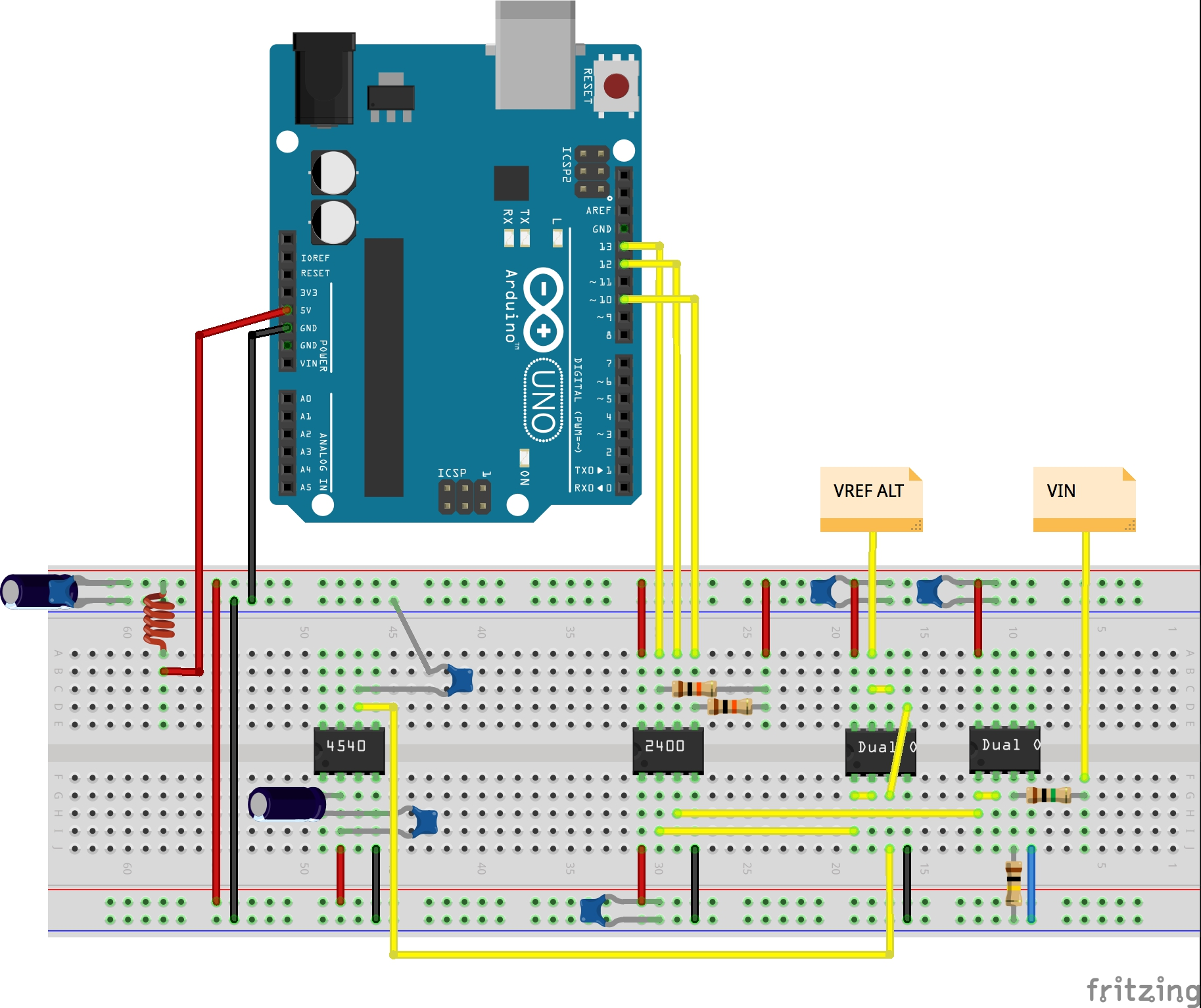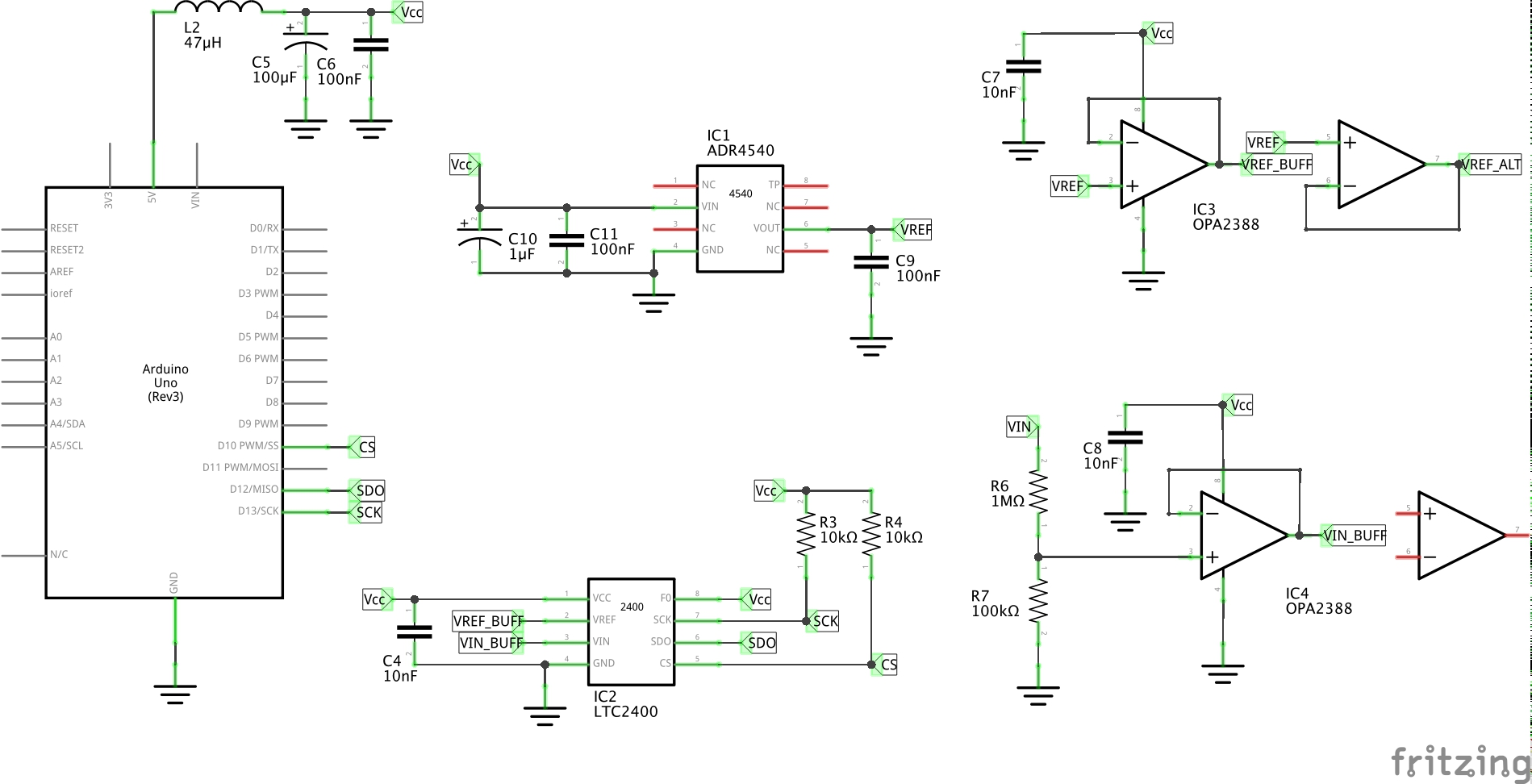## Conclusion - so far

Getting accurate readings with the LTC2400 takes a bit of work. A couple of things appear critical:

• buffering reference and input signals with a high-precision op-amp (such as the OPA2388)
• hefy bypass on each IC
• a good, stable power supply

So far I’ve got reasonable readings of a LT1019-2.5V reference voltage with the circuit on a breadboard. A 4.096V ADR4540 reference appears to offer slightly more accurate results.

Some things I’d like to try next:

• put the circuit on a PCB with a good ground plane